More Ways of Showing

These problems give you chance to find different ways of showing (or representing) your thinking.  They sit alongside the problems in our Ways of Showing Feature.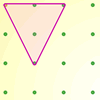Inside Triangles

Age 5 to 7 Challenge Level:

How many different triangles can you draw on the dotty grid which each have one dot in the middle?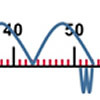Five Steps to 50

Age 5 to 7 Challenge Level:

Use five steps to count forwards or backwards in 1s or 10s to get to 50. What strategies did you use?Age 5 to 7 Challenge Level:

There are three baskets, a brown one, a red one and a pink one, holding a total of 10 eggs. Can you use the information given to find out how many eggs are in each basket?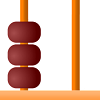Age 5 to 7 Challenge Level:

If you put three beads onto a tens/ones abacus you could make the numbers 3, 30, 12 or 21. What numbers can be made with six beads?A Bowl of Fruit

Age 5 to 7 Challenge Level:

Can you work out how many apples there are in this fruit bowl if you know what fraction there are?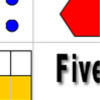Number Match

Age 5 to 11 Challenge Level:

A task which depends on members of the group noticing the needs of others and responding.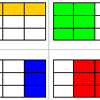Fraction Match

Age 5 to 11 Challenge Level:

A task which depends on members of the group noticing the needs of others and responding.Rectangle Tangle

Age 7 to 11 Challenge Level:

The large rectangle is divided into a series of smaller quadrilaterals and triangles. Can you untangle what fractional part is represented by each of the shapes?A4 Fraction Subtractionlive

Age 7 to 11 Challenge Level:

This task offers opportunities to subtract fractions using A4 paper.Chocolate

Age 7 to 14 Challenge Level:

There are three tables in a room with blocks of chocolate on each. Where would be the best place for each child in the class to sit if they came in one at a time?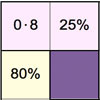Doughnut Percents

Age 7 to 14 Challenge Level:

A task involving the equivalence between fractions, percentages and decimals which depends on members of the group noticing the needs of others and responding.